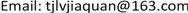﻿ 基于回归分析的城镇化发展对生态环境影响的研究——以Z市为例 Study on the Impact of Urbanization on Ecological Environment Based on Regression Analysis—Taking Z City as an Example

Statistics and Application
Vol. 08  No. 04 ( 2019 ), Article ID: 31858 , 7 pages
10.12677/SA.2019.84078

Study on the Impact of Urbanization on Ecological Environment Based on Regression Analysis

—Taking Z City as an Example

Jiaquan Lv, Xiaoyu Li, Yuxiang Zong, Wenyu Zhang, Xinyu Gao, Feng Hu

School of Statistics, Qufu Normal University, Qufu ShandongReceived: Aug. 2nd, 2019; accepted: Aug. 14th, 2019; published: Aug. 23rd, 2019ABSTRACT

The development of urbanization is an important factor to measure the comprehensive strength of a region. At the same time, the ecological environment is inextricably linked with people’s production and life. In order to study the impact of urbanization development on the ecological environment, this paper selects the relevant data of Z city, constructs the urbanization and ecological environment index system, takes the relevant indicators of urbanization development as the independent variables, and integrates multiple indicators related to the ecological environment through the entropy method. For the dependent variable, R software was used to analyze the sample data obtained, and the multiple regression equation was established by stepwise regression method. The analysis results show that factors such as per capita GDP have a positive impact on the ecological environment, and the amount of civilian vehicles has a negative impact on the ecological environment. Based on the above analysis results, this paper proposes rationalization opinions on urbanization development from the three aspects of population, economy and society, and promotes the harmonious development of ecological environment and urbanization.

Keywords:Urbanization, Ecological Environment, Entropy Method, Regression Analysis

——以Z市为例1. 引言

“城镇化”是指农村人口不断向城镇转移，第二、三产业不断向城镇聚集，从而使城镇数量增加、规模扩大的一种历史过程。城镇化问题是我国经济社会发展的重大问题，是“十二五”和“十三五”期间的重要任务。城镇经济建设与发展，必须注意合理利用资源保护生态环境，坚持可持续发展的方针，使得城镇的经济、社会和环境协调发展  。近年来，城镇化建设推动了我国经济社会的持续发展，促进了城乡居民生活水平的全面提升。但是，在城镇化取得卓越成就的同时，许多生态环境问题接踵而至，遏制了城镇化进程  。本文旨在通过多元统计分析、建立回归模型，初步研究城镇化建设对生态环境的影响，在不影响城镇化进程的前提下，提出改善农村生态环境的合理建议。

2. 理论依据 

2.1. 多元回归模型

$\left\{\begin{array}{l}y={\beta }_{0}+{\beta }_{1}{x}_{1}+{\beta }_{2}{x}_{2}+\cdots +{\beta }_{p}{x}_{p}+\epsilon \hfill \\ \epsilon ~N\left(0,{\sigma }^{2}\right)\hfill \end{array}$

2.2. 逐步回归

2.3. 熵权法的基本原理

3. 模型构建与结果分析

3.1. 指标体系的选取

3.2. 数据预处理

3.2.1. 数据标准化

3.2.2. 数据差分处理Table 1. Urbanization and ecological environment index systemFigure 1. Comparison of correlation coefficients before and after differential data

3.3. 因变量综合指标的提取

${y}_{ij}=\frac{{x}_{ij}-\mathrm{min}\left\{{x}_{1j},\cdots ,{x}_{nj}\right\}}{\mathrm{max}\left\{{x}_{1j},\cdots ,{x}_{nj}\right\}-\mathrm{min}\left\{{x}_{1j},\cdots ,{x}_{nj}\right\}}$

${p}_{ij}=\frac{{y}_{ij}}{\underset{i=1}{\overset{n}{\sum }}{y}_{ij}},i=1,\cdots ,n,j=1,\cdots ,m$

${E}_{j}=-\mathrm{ln}{\left(n\right)}^{-1}\underset{i=1}{\overset{n}{\sum }}{p}_{ij}\mathrm{ln}\left({p}_{ij}\right),j=1,\cdots ,m$

${d}_{j}=1-{E}_{j},j=1,\cdots ,m$

${\omega }_{j}=\frac{{d}_{j}}{\underset{j=1}{\overset{m}{\sum }}{d}_{j}},j=1,\cdots ,m$

3.4. 全模型Table 3. Full model coefficient and Variance expansion factor

$\Delta y=-3.911+1.365\Delta {x}_{1}+17.094\Delta {x}_{2}+0.612\Delta {x}_{3}+0.553\Delta {x}_{4}+1.960\Delta {x}_{5}-0.252\Delta {x}_{6}-3.472\Delta {x}_{7}-2.509\Delta {x}_{8}$

3.5. 逐步回归Table 4. Select model coefficient and variance expansion factor

$\Delta y=-3.457+0.961\Delta {x}_{1}+15.075\Delta {x}_{2}+0.334\Delta {x}_{4}+1.680\Delta {x}_{5}-2.735\Delta {x}_{7}-2.092\Delta {x}_{8}$

4. 相关建议

Study on the Impact of Urbanization on Ecological Environment Based on Regression Analysis—Taking Z City as an Example[J]. 统计学与应用, 2019, 08(04): 689-695. https://doi.org/10.12677/SA.2019.84078

1. 1. 王国惠, 赵新燕, 黄永胜. 新型城镇化与生态环境协调发展关系探究[J]. 经济问题, 2018(3): 112-117.

2. 2. 郭海红, 张在旭. 新型城镇化与生态环境响应度的区域差异研究[J]. 宁夏社会科学, 2018(6): 86-96.

3. 3. 史亚楠, 佟光霁. 浅析城镇化建设中生态环境保护的问题及对策[J]. 农业经济, 2013(8): 45-47.

4. 4. 何晓群, 刘文卿. 应用回归分析[M]. 第4版. 北京: 中国人民大学出版社, 2015: 132-157.